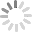# How to Create and Use Formulas in Excel

Formula is an Expression that actually calculates value of cell and Functions are predefined formulas that are already available in Excel. By using Formulas you can add manually, multiply, subtract values etc and can take more benefits from Excel Formulas and can make your work easier. The major difference between formula and function in Excel is that Formula is a user defined calculation While Function is a built-in calculation. If you are using a formula you can use a single function. Do you want to know how to create a formula in Excel? If yes, then read content below.

## Solutions on How to Create and Use Formula in Excel

Below Solutions will surely going to help you on how to use Excel formulas. Below guide is extremely helpful even a kindergarten student can implement these by following instructions carefully.

### Way 1: How to Create A Formula in Excel by Using Constants and Operators

Well, constants are dates, numbers or can be text values that we enter directly in a formula. Let's have a look below to simple Excel formula by using constants as well as operators.

Step 1: First you need to select the cell where you want to see the results.

Step 2: Now in formula bar you need to type equal (=) symbol and then you need to type the equation that you want to calculate.

Step 3: Now at the end you need to press Enter for proceeding formula.

Let's have a look below to simple Addition formula in Excel: =9+3.

### Way 2: How to Write A Formula in Excel by Using Defined Names

Well we have introduced amazing solution above on how to use Excel formulas, now let's have a look to create formula using defined names.

Step 1. First we need to create names for a certain cell. Fastest way to create names for cell is to select a cell and type the name directly in the Name Box.Step 2. After you have done with defining names you can type the following formula: =revenue-expenses### Way 3: How to Do Formulas in Excel Using Cell References

Cell reference is used to refer the cell. For example if you to subtract value in cell B2 from cell A2 then you need to write formula like that: =A2-B2. It's quite easy just type the formulas directly in the formula bar or you can select the cell, too.### Way 4: How to Make A Formula in Excel by Using Functions

As we have seen different solution for how to make a formula in Excel now let's have a look for making a formula in Excel by using functions.

Creating formula by using function is easy for example: =SUM(A1:A2) and SUM(A1,A2) both use the SUM function to add the values in cells A1 and A2. Formulas always start with an equal sign (=)

Step 1. First choose the cell where you want the formula. To proceed with formula by using function first you need to click in a formula bar and type your formula there.

Step 2. When you have done with formula arguments, then you need to press Enter.

Step 3. After pressing Enter you will be able to see the formula results in the cell that you selected in the start. It's done!

## Bonus Tips: How to Recover Forgotten Excel Password

Recovering forgotten Excel password is not a big deal now. PassFab for Excel is an awesome reliable tool 100% secure. Most of the users found unexpected errors while using other tools for recovering Excel password. But until now, this software has been tested by experts and they found it perfect. Its working is efficient as well as supports almost all versions.

### Instruction to Use PassFab for Excel

If you want to know how to recover Excel password, then this tool is the perfect match for your need. Let's have a look below on instructions to use this tool.

Step 1. If you have downloaded PassFab for Excel then install it. When you have done with downloading, such screen will appear you can see in the picture below.Step 2. Now you need to click Add button and import your file for which you are looking for password.Step 3. There you will be able to see three options. Just choose one of them and go ahead.

• First one is Dictionary Attack. Go to settings if you want to upload your own dictionary
• You can select second option, Brute Force with Mask, if you want to customize numbers or symbols.
• You can choose the third option, Brute Force. This option is made to try all possible combinations.Step 4. This is almost the end if you want to complete password recovery process do it by choosing "Start" button.Step 5. Congratutations! The lost Excel password is now recovered.As you have seen, this tool is so easy to use just few simple steps can takes you to have an access to your protected file.

## Summary